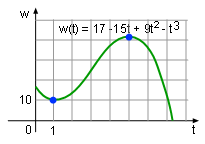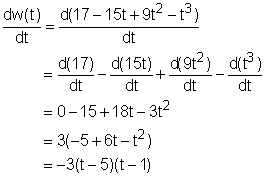Ch 4. The Mean Value Theorem Multimedia Engineering Math Maximum & Minimum Rolle'sTheorem Mean ValueTheorem MonotonicFunctions First DerivativeTest Concavity &Inflection SecondDerivative Test
 Chapter 1. Limits 2. Derivatives I 3. Derivatives II 4. Mean Value 5. Curve Sketching 6. Integrals 7. Inverse Functions 8. Integration Tech. 9. Integrate App. 10. Parametric Eqs. 11. Polar Coord. 12. Series Appendix Basic Math Units Search eBooks Dynamics Fluids Math Mechanics Statics Thermodynamics Author(s): Hengzhong Wen Chean Chin Ngo Meirong Huang Kurt Gramoll ©Kurt GramollMATHEMATICS - CASE STUDY SOLUTIONWork Function

Determining whether the expression, w(t) = 17 - 15t + 9t2 - t3, is the work function of an liver implant assist device.

The power should be positive for this liver implant assist device to work because the device is consuming power to generate energy. Recall that the derivative of the work function with respect to time is power, and thus the way to check whether the function is the proper work expression of this device is to figure out the sign of the power, in other words, the monotonicity of the work function. Overall, the derivative of the work expression needs to be calculated which will give an expression for power. That isThe critical point where the maximum and minimum energy may occur can be calculated by setting dw/dt to 0.

-3(t - 5)(t - 1) = 0

Solving this equation gives

t = 1 or t = 5

It is clear that the signs of the power, dw/dt, depends on two factors, t - 1 and t - 5. The domain of time is between 0 and +∞, which is broken down to three intervals by 1 and 5, they are (0, 1), (1, 5), and (5, +∞). The sign and the monotonicity are shown in the table. A "+" indicates a positive expression and a "-" means a negative expression.

 Interval Sign w t - 1 t - 5 dw/dt = -3(t - 5)(t - 1) 0 < t < 1 - - - decreasing on (0, 1) 1< t < 5 + - + increasing on (1, 5) 5 < t < 7 + + - decreasing on (5, 7)

According to the table, the interval for this device to work properly is between 1 and 5 years because in this period of time the sign for dw/dt is positive and the function is increasing. This makes no sense in the real life because the device does not work for the first year, but works for the following four years. Therefore, the expression is not the work function for the liver assist implant device.